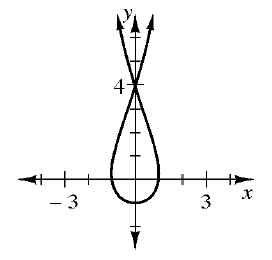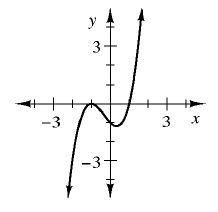### Home > INT1 > Chapter 1 > Lesson 1.2.2 > Problem1-48

1-48.

Find the $x$- and $y$-intercepts for the graphs of the relationships in problem 1-47.

See the graphs below from the previous problem. Read the $x$ and $y$ intercepts off the graphs and tables.

1.1. input $\boldsymbol {(x)}$

output $\boldsymbol {(y)}$

$-3$

$19$

$5$

$19$

$19$

$0$

$0$

$-3$

1.  input $\boldsymbol {(x)}$ output $\boldsymbol {(y)}$ $7$ $–2$ $0$ $7$ $4$ $10$ $0$ $10$ $3$ $0$
1.